## UGC NET CS 2015 Dec- paper-2

 Question 1
How many committees of five people can be chosen from 20 men and 12 women such that each committee contains at least three women?
 A 75240 B 52492 C 41800 D 9900
Engineering-Mathematics Engineering-Mathematics       Probability
Question 1 Explanation:
Given data,
-- 20 men and 12 women
-- 5 people can choose from men and women
-- Each committee contains at least three women
Step-1: We have a constraint that each committee contains atleast 3 women.
possibility-1: 2 men + 3 women.
possibility-2: 1 men + 4 women.
possibility-3: 0 men + 5 women.
Step-1: They are asking to find all possibilities.
= (possibility -1)+ (possibility -2) + (possibility -3)
=​ . ​ (​ 20​ C​ 2.​ *​ 12​ C​ 3​ ) + (​ 20​ C​ 2.​ *​ 12​ C​ 2​ ) + (​ 20​ C​ 2.​ *​ 12​ C​ 2​ )
= (190*220) + (20*495) + (1*792)
= 41800 + 9900 + 792
= 52492
 Question 2
Which of the following statement(s) is/are false ?
(a) A connected multigraph has an Euler Circuit if and only if each of its vertices has even degree.
(b) A connected multigraph has an Euler Path but not an Euler Circuit if and only if it has exactly two vertices of odd degree.
(c) A complete graph (K​ n​ ) has a Hamilton Circuit whenever n ≥ 3.
(d) A cycle over six vertices (C​ 6​ ) is not a bipartite graph but a complete graph over 3 vertices is bipartite.
 A (a) only B (b) and (c) C (c) only D (d) only
Engineering-Mathematics       Graph-Theory
Question 2 Explanation:
(a)TRUE: A connected multigraph has an Euler Circuit if and only if each of its vertices has even degree.
(b)TRUE: A connected multigraph has an Euler Path but not an Euler Circuit if and only if it has exactly two vertices of odd degree.
(c)TRUE: A complete graph (K​ n​ ) has a Hamilton Circuit whenever n ≥ 3. (d) FALSE: A cycle over six vertices (C​ 6​ ) is not a bipartite graph but a complete graph over 3 vertices is bipartite.
 Question 3
Which of the following is/are not true?
(a) The set of negative integers is countable.
(b) The set of integers that are multiples of 7 is countable.
(c)The set of even integers is countable.
(d)The set of real numbers between 0 and 1⁄2 is countable.
 A (a) and (c) B (b) and (d) C (b) only D (d) only
Engineering-Mathematics       Set-Theory
Question 3 Explanation:
(a)TRUE: The set of negative integers is countable.
Suppose negative integers set size is 10.
Ex: -1, -2, -3,.....,-10 is countable
(b)TRUE: The set of integers that are multiples of 7 is countable.
Suppose set of integers size is 10.
Ex: 1*7, 2*7, 3*7, .....,10*7 is countable
(c)TRUE: The set of even integers is countable.
Suppose set of even integers size is 10.
Ex: 2,4,6,8,10,....,20
(d) FALSE: The set of real numbers between 0 and 1⁄2 is countable. We can’t count real numbers.
Ex: 0.1, 0.2, 0.3, .....,0.∞
 Question 4Consider the graph given below: The two distinct sets of vertices, which make the graph bipartite are:
 A (v​ 1​ , v​ 4​ , v​ 6​ ); (v​ 2​ , v​ 3​ , v​ 5​ , v​ 7​ , v​ 8​ ) B (v​ 1​ , v​ 7​ , v​ 8​ ); (v​ 2​ , v​ 3​ , v​ 5​ , v​ 6​ ) C (v​ 1​ , v​ 4​ , v​ 6​ , v​ 7​ ); (v​ 2​ , v​ 3​ , v​ 5​ , v​ 8​ ) D (v​ 1​ , v​ 4​ , v​ 6​ , v​ 7​ , v​ 8​ ); (v​ 2​ , v​ 3​ , v​ 5​ )
Engineering-Mathematics       Graph-Theory
Question 4 Explanation:
A bipartite graph (or bigraph) is a graph whose vertices can be divided into two disjoint and independent sets U and V such that every edge connects a vertex in U to one in V. Vertex sets U and V are usually called the parts of the graph. Equivalently, a bipartite graph is a graph that does not contain any odd-length cycles.
→ The two sets U and V may be thought of as a coloring of the graph with two colors.
Option A: FALSE because V​ 5​ , V​ 7​ and V​ 3​ are adjacent. So, it not not bipartite graph.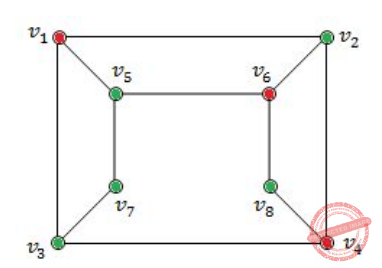Option-B FALSE because V​ 5​ , V​ 6​ and V​ 2​ are adjacent. So, it not not bipartite graph.Option-C TRUE because it follows properties of bipartied and no two colours are adjacent.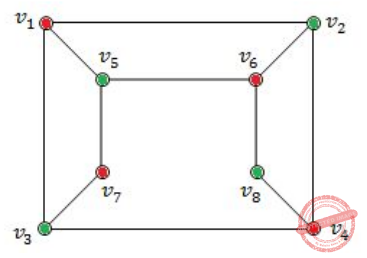Option-D FALSE because because V​ 4​ , V​ 6​ and V​ 8​ are adjacent. So, it not not bipartite graph.Question 5
A tree with n vertices is called graceful, if its vertices can be labelled with integers 1, 2,....n such that the absolute value of the difference of the labels of adjacent vertices are all different. Which of the following trees are graceful?
(a)(b)(c)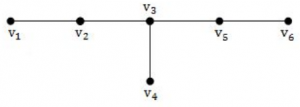A (a) and (b) B (b) and (c) C (a) and (c) D (a), (b) and (c)
Engineering-Mathematics       Graph-Theory
Question 5 Explanation:
Above all graphs are graceful.Question 6
Which of the following arguments are not valid ?
(a) “If Gora gets the job and works hard, then he will be promoted. If Gora gets promotion, then he will be happy. He will not be happy, therefore, either he will not get the job or he will not work hard”.
(b) “Either Puneet is not guilty or Pankaj is telling the truth. Pankaj is not telling the truth, therefore, Puneet is not guilty”.
(c) If n is a real number such that n >1, then n​ 2 ​ >1. Suppose that n​ 2 ​ >1, then n >1.
 A (a) and (c) B (b) and (c) C (a), (b) and (c) D (a) and (b)
Engineering-Mathematics       Combinatorics
 Question 7
Let P(m, n) be the statement “m divides n” where the Universe of discourse for both the variables is the set of positive integers. Determine the truth values of the following propositions.
(a)∃m ∀n P(m, n)
(b)∀n P(1, n)
(c) ∀m ∀n P(m, n)
 A (a) - True; (b) - True; (c) - False B (a) - True; (b) - False; (c) - False C (a) - False; (b) - False; (c) - False D (a) - True; (b) - True; (c) - True
Artificial-intelligence       Propositional-Logic
Question 7 Explanation:
Given P(m,n) ="m divides n"
Statement-A is ∃m ∀n P(m, n). Here, there exists some positive integer which divides every positive integer. It is true because there is positive integer 1 which divides every positive integer.
Statement-B is ∀n P(1, n). Here, 1 divided every positive integer. It is true.
Statement-C is ∀m ∀n P(m, n). Here, every positive integer divided every positive integer. It is false.
 Question 8
Match the following terms:A (i)(ii)(iii)(iv) B (ii)(iii)(i)(iv) C (iii)(ii)(iv)(i) D (iv)(iii)(ii)(i)
Engineering-Mathematics       Propositional-Logic
Question 8 Explanation:
→ Vacuous proof is a proof that the implication p → q is true based on the fact that p is false.
→ Trivial proof is a proof that the implication p → q is true based on the fact that q is true.
→ Direct proof is a proof that the implication p → q is true that proceeds by showing that q must be true when p is true.
→ Indirect proof is a proof that the implication p → q is true that proceeds by showing that p must be false when q is false.
 Question 9
Consider the compound propositions given below as:
(a)p ∨ ~(p ∧ q)
(b)(p ∧ ~q) ∨ ~(p ∧ q)
(c)p ∧ (q ∨ r)
Which of the above propositions are tautologies?
 A (a) and (c) B (b) and (c) C (a) and (b) D only (a)
Engineering-Mathematics       Propositional-Logic
Question 9 Explanation: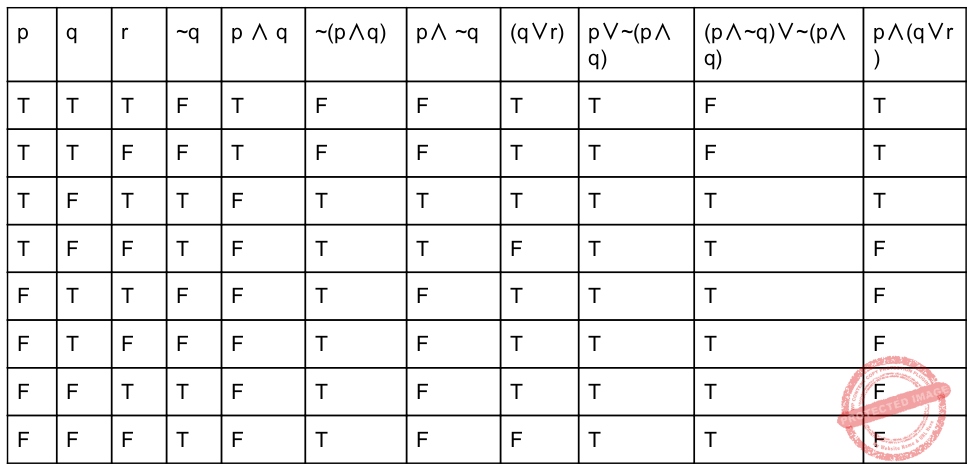Question 10
Which of the following property/ies a Group G must hold, in order to be an Abelian group?
(a)The distributive property
(b)The commutative property
(c)The symmetric property
 A (a) and (b) B (b) and (c) C (a) only D (b) only
Engineering-Mathematics       Set-Theory
Question 10 Explanation:
An abelian group is a set, A, together with an operation • that combines any two elements a and b to form another element denoted a • b. The symbol • is a general placeholder for a concretely given operation. To qualify as an abelian group, the set and operation, (A, •), must satisfy five requirements known as the abelian group axioms:
Closure: For all a, b in A, the result of the operation a • b is also in A.
Associativity: For all a, b and c in A, the equation (a • b) • c = a • (b • c) holds.
Identity element: There exists an element e in A, such that for all elements a in A, the equation e • a = a • e = a holds.
Inverse element: For each a in A, there exists an element b in A such that a • b = b • a = e, where e is the identity element.
Commutativity: For all a, b in A, a • b = b • a.
A group in which the group operation is not commutative is called a "non-abelian group" or "non-commutative group".
 Question 11
Consider the following program :
#include
main( )
{
int i, inp;
float x, term=1, sum=0;
scanf(“%d %f ”, &inp, &x);
for(i=1; i<=inp; i++)
{
term = term * x/i;
sum = sum + term ;
}
printf(“Result = %f\n”, sum);
}
The program computes the sum of which of the following series?
 A x + x​ 2​ /2 + x​ 3​ /3 + x​ 4​ /4 +... B x + x​ 2​ /2! + x​ 3​ /3! + x​ 4​ /4! +... C 1 + x​ 2​ /2 + x​ 3​ /3 + x​ 4​ /4 +... D 1 + x​ 2​ /2! + x​ 3​ /3! + x​ 4​ /4! +...
Programming       Programming
Question 11 Explanation:
In this problem, we have to find series of evaluation.
Let x=5, inp=5
Iteration-1:
for(i=1; i<=inp; i++)
/* condition true 1<=5 */
{
term = term * x/i;
/* (1*5)/1=5. Here, 5 nothing but ‘x’ */
sum = sum + term ; /* 0+5=5 */
}
Iteration-2: Here, ‘i’ becomes 2.
for(i=2; i<=inp; i++)
/* condition true 2<=5 */
{
term = term * x/i;
/* (5*5)/2 is nothing but x​ 2​ /i */
sum = sum + term ; /* 5+12=17 */
}
;;;
;;;
Iteration-5: Here, ‘i’ becomes 5.
for(i=5; i<=inp; i++)
/* condition true 5<=5 */
{
term = term * x/i;
/* (625*5)/5! is nothing but x​ 5​ /i! */
sum = sum + term ;
}
 Question 12
Consider the following two statements:
(a) A publicly derived class is a subtype of its base class.
(b) Inheritance provides for code reuse.
 A Both the statements (a) and (b) are correct. B Neither of the statements (a) and (b) are correct C Statement (a) is correct and (b) is incorrect D Statement (a) is incorrect and (b) is correct.
OOPS       Inheritance
Question 12 Explanation:
→ A publicly derived class is a subtype of its base class.
→ Inheritance is defined as deriving new classes (sub classes) from existing ones (super class or base class) and forming them into a hierarchy of classes.
→ Inheritance allows programmers to create classes that are built upon existing classes, to specify a new implementation while maintaining the same behaviors (realizing an interface), to reuse code and to independently extend original software via public classes and interfaces.
 Question 13
Consider a “CUSTOMERS” database table having a column “CITY” filled with all the names of Indian cities (in capital letters). The SQL statement that finds all cities that have “GAR” somewhere in its name, is:
 A Select * from customers where city = ‘%GAR%’; B Select * from customers where city = ‘\$GAR\$’; C Select * from customers where city like ‘%GAR%’; D Select * from customers where city as ‘%GAR’;
Database-Management-System       SQL
Question 13 Explanation:
In above question a specific pattern "GAR" is given for pattern matching.
In SQL "LIKE" clause is used for pattern matching. For LIKE clause we have two wild cards:
1. "%" which represents any sequence of "0" or more characters.
2. "_" is used to replace a single character.
So, Option C is the correct answer because they have used LIKE clause along with "%" which indicates any number of character can be present before and after "GAR" pattern.
 Question 14
Match the following database terms to their function:A (iv)(iii)(i)(ii) B (ii)(iv)(i)(iii) C (ii)(iv)(iii)(i) D (iv)(iii)(ii)(i)
Database-Management-System       Match-the-following
Question 14 Explanation:
Normalization: Normalization is used to reduce the data redundancy in the database
Data Dictionary: Data dictionary contains meta describing database structure.
Referential Integrity: Referential integrity enforces match of primary key to foreign key
External Schema: Define view(s) of the database for particular user(s)
 Question 15
In general, in a recursive and non-recursive implementation of a problem (program) :
 A Both time and space complexities are better in recursive than in non-recursive program. B Both time and space complexities are better in non-recursive than in recursive program C Time complexity is better in recursive version but space complexity is better in non-recursive version of the program. D Space complexity is better in recursive version but time complexity is better in non-recursive version of the program.
Algorithms       Time-Complexity
Question 15 Explanation:
Both time and space complexities are better in non-recursive than in recursive program. We can also call non-recursive programs are iterative programs. Iterative programs time and space complexity is better than recursive program.
→ Recursion uses more memory, but is sometimes clearer and more readable. Using loops increases the performance, but recursion can sometimes be better for the programmer (and his performance). Depends on what we you want to implement, and what's more important for you (readability, performance...)
Example: Factorial of a number.
 Question 16
A three dimensional array in ‘C’ is declared as int A[x][y][z]. Here, the address of an item at the location A[p][q][r] can be computed as follows (where w is the word length of an integer):
 A &A + w(y* z* q + z* p + r) B &A + w(y* z* p + z*q + r) C &A + w(x* y* p + z* q+ r) D &A + w(x* y* q + z* p + r)
Data-Structures       Arrays
 Question 17
In C++, which system - provided function is called when no handler is provided to deal with an exception?
 A terminate( ) B unexpected( ) C abort( ) D kill( )
OOPS       Programming-in-c++
Question 17 Explanation:
In some cases, the exception handling mechanism fails and a call to void terminate() is made. This terminate() call occurs in any of the following situations:
The exception handling mechanism cannot find a handler for a thrown exception. The following cases are more specific:
1. During stack unwinding, a destructor throws an exception and that exception is not handled.
2. The expression that is thrown also throws an exception, and that exception is not handled.
3. The constructor or destructor of a nonlocal static object throws an exception, and the exception is not handled.
4. A function registered with atexit() throws an exception, and the exception is not handled. The following demonstrates this:
A throw expression without an operand tries to rethrow an exception, and no exception is presently being handled.
 Question 18
Which of the following provides the best description of an entity type?
 A A specific concrete object with a defined set of processes (e.g. Jatin with diabetes) B A value given to a particular attribute (e.g. height - 230 cm) C A thing that we wish to collect data about zero or more, possibly real world examples of it may exist D A template for a group of things with the same set of characteristics that may exist in the real world
Database-Management-System       ER-Model
Question 18 Explanation:
A template for a group of things with the same set of characteristics that may exist in the real world is best description of entity type.
Except option-D, remaining specifying their attributes.
 Question 19
Data which improves the performance and accessibility of the database are called:
 A Indexes B User Data C Application Metadata D Data Dictionary
Database-Management-System       Constraints
Question 19 Explanation:
→ An index is a data which improves the performance and accessibility of the database. An index is a copy of selected columns of data from a table that can be searched very efficiently that also includes a low-level disk block address or direct link to the complete row of data it was copied from.
→ To create the application metadata XML file containing details of all the Business Process Server applications that need to be packaged and published.
→ A data dictionary (or) metadata repository is a "centralized repository of information about data such as meaning, relationships to other data, origin, usage, and format".
 Question 20
A relation R = {A, B, C, D, E, F,G} is given with following set of functional dependencies: F = {AD → E, BE → F, B → C, AF → G} Which of the following is a candidate key ?
 A A B AB C ABC D ABD
Database-Management-System       Functional-Dependency
Question 20 Explanation:
Since no functional dependency is having ABD in their right hand side, so every key should include ABD as a part of them to become a candidate key.
Now check whether ABD is itself a candidate key or not by finding the closure.
(ABD)​ +​ = { ABDCEFG }
since ABD can identify each attribute of the given relation uniquely.
So, ABD is the candidate key.
 Question 21
Which of the following services is not provided by wireless access point in 802.11 WLAN ?
 A Association B Disassociation C Error correction D Integration
Data-Communication       WLAN
Question 21 Explanation:
→ Wireless LANs are use high frequency radio waves instead of cables for connecting the devices in LAN. Users connected by WLANs can move around within the area of network coverage. Most WLANs are based upon the standard IEEE 802.11 or WiFi.
→ A wireless access point (WAP), or more generally just access point (AP), is a networking hardware device that allows other Wi-Fi devices to connect to a wired network.
→ Wireless access point in 802.11 WLAN services are association, association and Integration.
 Question 22
Which of the following fields in IPv4 datagram is not related to fragmentation?
 A Type of service B Fragment offset C Flags D Identification
Computer-Networks       IPv4-and-Fragmentation
Question 22 Explanation:
→ The Identification field along with the foreign and local internet address and the protocol ID.
→ Fragment offset field along with Don't Fragment and More Fragment flags in the IP protocol header are used for fragmentation and reassembly of IP packets.
→ The More Fragment(MF) flag is set for all the fragment packets except the last one.
 Question 23
Four channels are multiplexed using TDM. If each channel sends 100 bytes/second and we multiplex 1 byte per channel, then the bit rate for the link is __________.
 A 400 bps B 800 bps C 1600 bps D 3200 bps
Data-Communication       Multiplexing
Question 23 Explanation:
Given data,
-- 4 channels are multiplexed using TDM
-- Multiplex 1 byte per channel. So, total 4 bytes=4 channels.
-- Each channel sends 100 bytes/sec
-- Bit rate=?
Bit rate= 100 * 4 bytes(1 byte=8 bits)
= 100 * 4 *8 (we are converting into bits because we are finding bit rate)
= 3200 bps
 Question 24
In a typical mobile phone system with hexagonal cells, it is forbidden to reuse a frequency band in adjacent cells. If 840 frequencies are available, how many can be used in a given cell?
 A 280 B 110 C 140 D 120
Data-Communication       Frequency-Band
Question 24 Explanation:
-- Mobile phone system with hexagonal( equals to 6) cells
-- 840 frequencies are available.
-- How many can be used in a cell?
In this we need maximum 3 unique cells are required.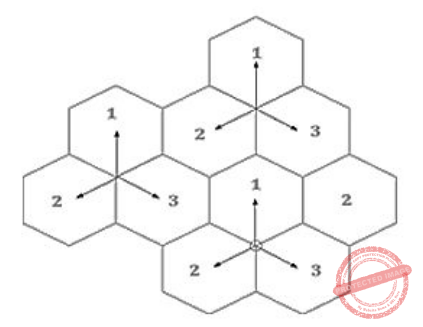Total cells used with frequency is = 840/3
= 280 frequencies
 Question 25
Using p = 3, q = 13, d = 7 and e = 3 in the RSA algorithm, what is the value of ciphertext for a plain text 5 ?
 A 8 B 16 C 26 D 33
\"Computer-Networks \"       RSA-algorithm
Question 25 Explanation:
Step-1: p=3, q=13, d=7 and e=3
n=p*q [n is the modulus for the public key and the private keys]
=39
Step-2: Calculate the totient: φ(n)= (p−1)*(q−1)
= 2*12
= 24
Step-3: Find cipher text we have an formula
C = M​ e​ mod n
= 5​ 3​ mod 39
= 125 mod 39
= 8
 Question 26
A virtual memory has a page size of 1K words. There are eight pages and four blocks. The associative memory page table contains the following entries:Which of the following list of virtual addresses (in decimal) will not cause any page fault if referenced by the CPU ?
 A 1024, 3072, 4096, 6144 B 1234, 4012, 5000, 6200 C 1020, 3012, 6120, 8100 D 2021, 4050, 5112, 7100
Operating-Systems       Virtual Memory
Question 26 Explanation:
Step-1: Total number of blocks=4
Total number of pages=8
Virtual memory has a page size of 1K(1024) words.
Step-2: Page ranges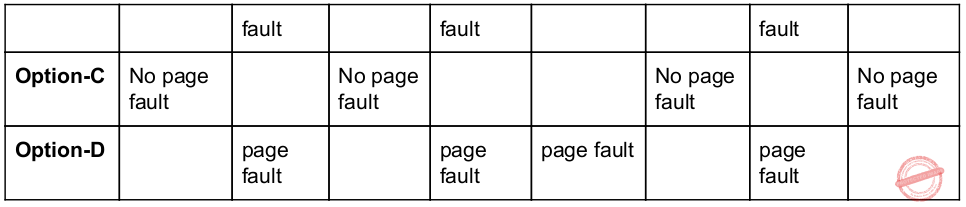Question 27
Suppose that the number of instructions executed between page fault is directly proportional to the number of page frames allocated to a program. If the available memory is doubled, the mean interval between page faults is also doubled. Further, consider that a normal instruction takes one microsecond, but if a page fault occurs, it takes 2001 microseconds. If a program takes 60 sec to run, during which time it gets 15,000 page faults, how long would it take to run if twice as much memory were available?
 A 60 sec B 30 sec C 45 sec D 10 sec
Operating-Systems       Page-fault
Question 27 Explanation:
Normal instruction takes 1 microsecond = 10-6 sec
→ Instruction with page fault takes 2001 microseconds so page fault takes additional 2000 microsecond
→ The given program takes 60 sec to run and in that program there were 15000 p.f
→ Since time taken by 1 page fault is 2000 microseconds, time taken by all 15000 page faults = 15000*2000 microseconds
=30 sec
→ Out of overall 60 sec of the program time 30 sec are taken for page faults, remaining 30 sec are consumed by program execution.
→ If memory is doubled we will have more memory available to hold the pages and hence page faults also reduce. It is given in the question that "if memory is doubled then the mean interval between the page faults is also doubled" this is nothing but "number of page faults will be reduced by half".
→ Earlier 30 sec were needed by program instructions and 30 sec for page faults.
→ Now program execution takes 30 sec and page fault will take only 15 sec.
So new total time =30+15
=45 sec
 Question 28
Consider a disk with 16384 bytes per track having a rotation time of 16 msec and average seek time of 40 msec. What is the time in msec to read a block of 1024 bytes from this disk?
 A 57 sec B 49 sec C 48 sec D 17 sec
Operating-Systems       Rotational-latency
Question 28 Explanation:
Given data,
-- Bytes per track=16384
-- Rotational time=16 msec.
-- Average seek time= 40 msec
-- What is the time in msec to read a block of 1024 bytes from this disk?
Step-1: Total time= Seek Time + Rotational Latency + Transfer Time = ? + ? + 40 msec
Step-2: Transfer time = ((1024 bytes *16 ms)/ (16384 bytes )) = 1 msec
Step-3: Rotational latency = 16 msec / 2
= 8 msec
Step-4: Total time= Seek Time + Rotational Latency + Transfer Time
= 40+8+1 msec
= 49 msec
 Question 29
A system has four processes and five allocatable resources. The current allocation and maximum needs are as follows: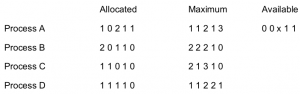The smallest value of ‘x’ for which the above system in safe state is __________.
 A 1 B 3 C 2 D Not safe for any value of x.
Question 29 Explanation:Case 1: Let’s consider x=1
→ Now need of Process D can be fulfilled. After completing its execution Process D leaves its allocated resources.
→ Now Need of any either Process can’t be fulfilled. So the system is not in safe state with x=1.
Case 2: x=2
Available = 0 0 2 1 1
→ Need of Process D can be fulfilled and after its execution it release its allocated resources.
Available
= 0 0 2 1 1
D
= 1 1 1 1 0
---------------------------------- New available = 1 1 2 2 1
→ Now Need of Process ‘C’ can be fulfilled.
Available
= 1 1 3 2 1
C
= 1 1 0 1 0
----------------------------------
New available = 2 2 3 3 1
→ Now Need of Process ‘B’ can be fulfilled. So new available resources are
Available
= 2 2 3 3 1
C
= 2 0 1 1 0
----------------------------------
New available = 4 2 4 4 1
→ But now need of Process ‘A’ can’t be fulfilled with above available resources. So the system is not in safe state with x=2.
Case 3: x=3
Available = 0 0 3 1 1
→ Now Need of Process ‘D’ can be fulfilled and after its execution it will release its allocated resources.
Available
= 0 0 3 1 1
D
= 1 1 1 1 0
----------------------------------
New available = 1 1 4 2 1
→ Now Need of Process ‘C’ can be fulfilled
Available
= 1 1 4 2 1
C
= 1 1 0 1 0
----------------------------------
New available = 2 2 4 3 1
→ Now Need of Process ‘B’ can be fulfilled
Available
= 2 2 4 3 1
B
= 2 0 1 1 0
----------------------------------
New available = 4 2 5 4 1
→ But with available resource need of Process ‘A’ can’t be fulfilled. So the system is not in safe state with x=3.
→ Hence option (D) is the correct answer.
 Question 30
In Unix, the login prompt can be changed by changing the contents of the file __________
 A contrab B init C gettydefs D inittab
Operating-Systems       UNIX
Question 30 Explanation:
gettydefs​ : ​ speed and tty settings used by getty. The file /etc/gettydefs contains information used by getty(1m) to set up the speed and tty settings for a line. It supplies information on what the login-prompt should look like. It also supplies the speed to try next if the user indicates the current speed is not correct by typing a character.
init​ (short for initialization) is the first process started during booting of the computer system. Init is a daemon process that continues running until the system is shut down.
inittab​ - format of the inittab file used by the sysv-compatible init process. The inittab file describes which processes are started at bootup and during normal operation (e.g. /etc/init.d/boot, /etc/init.d/rc, gettys...). Init(8) distinguishes multiple runlevels, each of which can have its own set of processes that are started. Valid runlevels are 0-6 plus A, B, and C for on demand entrie.
crontab​ (short for "cron table") is a list of commands that are scheduled to run at regular time intervals on your computer system. The crontab command opens the crontab for editing, and lets you add, remove, or modify scheduled tasks.
 Question 31
A data cube C, has n dimensions, and each dimension has exactly p distinct values in the base cuboid. Assume that there are no concept hierarchies associated with the dimensions. What is the maximum number of cells possible in the data cube, C?
 A p​ n B p C (2​ n​ - 1)p+1 D (p + 1)​ n
Data-Communication       Mobile-Computing
Question 31 Explanation:
Option-A:​ This is the maximum number of distinct tuples that you can form with p distinct values per dimensions.
Option-B:​ You need at least p tuples to contain p distinct values per dimension. In this case no tuple shares any value on any dimension.
Option-C:​ The minimum number of cells is when each cuboid contains only p cells, except for the apex, which contains a single cell.
Option-D:​ We have p+1 because in addition to the p distinct values of each dimension we can also choose ∗.
 Question 32
Suppose that from given statistics, it is known that meningitis causes stiff neck 50% of the time, that the proportion of persons having meningitis is 1 / 50000 , and that the proportion of people having stiff neck is 1 / 20. Then the percentage of people who had meningitis and complain about stiff neck is:
 A 0.01% B 0.02% C 0.04% D 0.05%
Engineering-Mathematics       Statistics
 Question 33
__________ system is market oriented and is used for data analysis by knowledge workers including Managers, Executives and Analysts.
 A OLTP B OLAP C Data System D Market System
Database-Management-System       Data-models
Question 33 Explanation:
→ OLAP system is market oriented and is used for data analysis by knowledge workers including Managers, Executives and Analysts.
→ OLAP include business reporting for sales, marketing, management reporting, business process management (BPM), budgeting and forecasting, financial reporting and similar areas, with new applications emerging, such as agriculture.
 Question 34
__________ allows selection of the relevant information necessary for the data warehouse.
 A The Top - Down View B Data Warehouse View C Datasource View D Business Query View
Database-Management-System       Data-ware-housing
Question 34 Explanation:
→ ​ Top-Down​ is also known as Inmon’s Top Down Approach, data warehouse is built first. Inmon defines a data warehouse as a centralized repository for the entire enterprise. Dimensional data marts are created only after the complete data warehouse has been created.
→ ​ Bottom-Up​ is also called as Kimball’s bottom up approach, the most important business aspects or departments, data marts are created first. A data mart provide a thin view into the organisational data and addresses a single business area. These data marts are then integrated into larger data warehouse to build a complete data warehouse. The integration of data marts is implemented using Kimball’s data warehousing architecture which is also known as data warehouse bus (BUS).
 Question 35
The hash function used in double hashing is of the form:
 A h (k, i) = (h​ 1​ (k) + h​ 2​ (k) + i) mod m B h (k, i) = (h​ 1​ (k) + h​ 2​ (k) - i) mod m C h (k, i) = (h​ 1​ (k) + i h​ 2​ (k)) mod m D h (k, i) = (h​ 1​ (k) - i h​ 2​ (k)) mod m
Data-Structures       Hashing
Question 35 Explanation:
Double Hashing with second hash function is​ ​ h(k,i) = (h​ 1​ (k) + i·h​ 2​ (k)) mod m 0≤ i ≤m-1
h​ 1​ → hash function
h​ 2​ → Step function
First try h(k,0) = h​ 1​ (k), if it is occupied, try h(k,1) etc..,
 Question 36
In the following graph, discovery time stamps and finishing time stamps of Depth First Search (DFS) are shown as x/y, where x is discovery time stamp and y is finishing time stamp.It shows which of the following depth first forest?
 A {a, b, e} {c, d, f, g, h} B {a, b, e} {c, d, h} {f, g} C {a, b, e} {f, g} {c, d} {h} D {a, b, c, d} {e, f, g} {h}
Algorithms       Depth-first-search
Question 36 Explanation:
A DFS starting at some vertex ‘v’ explores the graph by building up a tree that contains all vertices that are reachable from ‘v’ and all edges that are used to reach these vertices. We call this tree a DFS tree. A complete DFS exploring the full graph (and not only the part reachable from a given vertex ‘v’) builds up a collection of trees, or forest, called a DFS forest.
Based on definition of DFS forest, option-A is correct.
 Question 37
The number of disk pages access in B-Tree search, where ‘h’ is height, ‘n’ is the number of keys, and ‘t’ is the minimum degree, is:
 A θ(log​ n​ h*t) B θ(log​ t​ n*h) C θ(log​ h​ n) D θ(log​ t​ n)
Data-Structures       B-Trees
Question 37 Explanation:
The number of disk pages access in B-Tree search, where ‘h’ is height, ‘n’ is the number of keys, and ‘t’ is the minimum degree, is θ(log​ t​ n).
Note: B-Tree search operation best,average and worst case will take θ(logn).
 Question 38
The inorder traversal of the following tree is:A 2 3 4 6 7 13 15 17 18 18 20 B 20 18 18 17 15 13 7 6 4 3 2 C 15 13 20 4 7 1718 2 3 6 18 D 2 4 3 13 7 6 15 17 20 18 18
Data-Structures       Tree-traversals
Question 38 Explanation:
Inorder traversal through Left, Root and Right. Inorder yield procedure given below.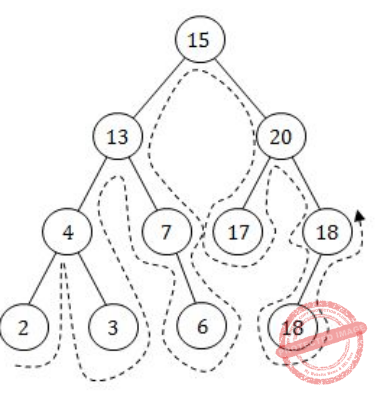Question 39
An ideal sort is an in-place-sort whose additional space requirement is __________.
 A O (log​ 2​ n) B O (n log​ 2​ n) C O (1) D O (n)
Algorithms       Sorting
Question 39 Explanation:
The ideal sorting algorithm would have the following properties:
1. Stable: Equal keys aren't reordered.
2. Operates in place, requiring O(1) extra space.
3. Worst case O(nlog(n)) key comparisons.
4. Worst case O(n) swaps.
5. Adaptive: Speeds up to O(n) when data is nearly sorted or when there are few unique keys.
 Question 40
Which of the following is not a congestion policy at network layer?
 A Flow Control Policy B Packet Discard Policy C Packet Lifetime Management Policy D Routing Algorithm
Computer-Networks       Which of the following is not a congestion policy at network layers
Question 40 Explanation:
Network Layer congestion policies:
1.Preallocation of Resources
2.Traffic Shaping
4.Isarithmic Congestion Control
6.Choke Packets
9. Routing Algorithm
Transport layer congestion policies:
1. Flow Control, etc..,
 Question 41
Loop unrolling is a code optimization technique:
 A that avoids tests at every iteration of the loop. B that improves performance by decreasing the number of instructions in a basic block. C that exchanges inner loops with outer loops D that reorders operations to allow multiple computations to happen in parallel
Compiler-Design       Code-Optimization
Question 41 Explanation:
→ Loop unrolling is a code optimization technique that avoids tests at every iteration of the loop.
→ The goal of loop unwinding is to increase a program's speed by reducing (or) eliminating instructions that control the loop, such as pointer arithmetic and "end of loop" tests on each iteration.
 Question 42
What will be the hexadecimal value in the register ax (32-bit) after executing the following instructions?
mov al, 15
mov ah, 15
xor al, al
mov cl, 3
shr ax, cl
 A 0F00 h B 0F0F h C 01E0 h D FFFF h
Computer-Organization       8085-Microprocessor
Question 42 Explanation:
Step-1: mov al, 15 ← Move 15 to lower part of 'ax' register
Step-2: mov ah, 15 ← Move 15 to higher part of 'ax' register
Step-3: xor al,al ← Perform Xor operation.
convert 15 into binary number is 00001111
00001111 ← al
00000000 ← al
-------------
00000000
--------------
Step-4: move cl,3 ← Move 3 to lower part of 'cx' register
Step-5: shr ax, cl ← It will shift right and rotate content of 'ax' register
Before shifting ‘ax’ value is 0000 1111 0000 0000
After 3 shift right operations then ‘ax’ will become 0000 0001 1110 0000
The value is nothing but 01E0 h (or) (01E0)​ 16
 Question 43
Which of the following statements is false?
 A Top-down parsers are LL parsers where first L stands for left - to - right scan and second L stands for a leftmost derivation. B (000)* is a regular expression that matches only strings containing an odd number of zeroes, including the empty string. C Bottom-up parsers are in the LR family, where L stands for left - to - right scan and R stands for rightmost derivation. D The class of context - free languages is closed under reversal. That is, if L is any context- free language, then the language L​ R​ = {w​ R​ : w∈L} is context - free.
Computer-Organization       Parsers
Question 43 Explanation:
TRUE: Top-down parsers are LL parsers where first L stands for left - to - right scan and second L stands for a leftmost derivation.
FALSE: (000)* is a regular expression that matches any string containing at least 3 number of zeroes, including the empty string.
TRUE: Bottom-up parsers are in the LR family, where L stands for left - to - right scan and R stands for rightmost derivation.
TRUE: The class of context - free languages is closed under reversal. That is, if L is any context - free language, then the language L​ R​ = {w​ R​ : w∈L} is context - free.
 Question 44
System calls are usually invoked by using :
 A A privileged instruction B An indirect jump C A software interrupt D Polling
Operating-Systems       System-Calls
Question 44 Explanation:
→ System calls are usually invoked by using a software interrupt.
→ Software interrupts are implementing device drivers (or) transitions between protected mode of operations, such as system calls.
 Question 45
The __________ transfers the executable image of a C++ program from hard disk to main memory.
Programming       Programming-in-c++
Question 45 Explanation:
→ The loader transfers the executable image of a C++ program from hard disk to main memory.
→ Loader is the program of the operating system which loads the executable from the disk into the primary memory(RAM) for execution. It allocates the memory space to the executable module in main memory and then transfers control to the beginning instruction of the program .
→ Loading a program involves reading the contents of the executable file containing the program instructions into memory, and then carrying out other required preparatory tasks to prepare the executable for running. Once loading is complete, the operating system starts the program by passing control to the loaded program code.
 Question 46
In software testing, how the error, fault and failure are related to each other?
 A Error leads to failure but fault is not related to error and failure. B Fault leads to failure but error is not related to fault and failure. C Error leads to fault and fault leads to failure. D Fault leads to error and error leads to failure.
Software-Engineering       Software-testing
Question 46 Explanation:
→ Error: A human action that produces an incorrect result. This is also sometimes referred to as Mistake.
→ Fault: A manifestation of an error in software, also known as Defect or Bug.
→ Failure: A deviation of the software from its expected delivery or service.
Relation between Error,Fault and FailureQuestion 47
Which of the following is not a software process model?
 A Prototyping B Iterative C Time-boxing D Glass-boxing
Software-Engineering       Software-process-models
Question 47 Explanation:
Software process models of Prototype and Iterative we know before also.
Time boxing model:
The development is done iteratively as in the iterative enhancement model and each iteration is done in a timebox of fixed duration. The functionality to be developed is adjusted to fit the duration of the timebox. Moreover, each timebox is divided into a sequence of fixed stages where each stage performs a clearly defined task (analysis, implementation, and deploy) that can be done independently. This model also requires that the time duration of each stage is approximately equal so that pipelining concept is employed to have the reduction in development time and product releases.
 Question 48
How many solutions are there for the equation x + y + z + u = 29 subject to the constraints that x ≥ 1, y ≥ 2, z ≥ 3 and u ≥ 0?
 A 4960 B 2600 C 23751 D 8855
Engineering-Mathematics       Permutations-combinations
Question 48 Explanation:
Given data,
-- The equation x+y+z+u = 29
-- Constraints are x ≥ 1, y ≥ 2, z ≥ 3 and u ≥ 0
-- Possible solutions?
Step-1: Combine all constraints (1+2+3+0) =6
Step-2: Subtract all constraints values with total equation number 29-6 =23.
Possibility to get repetition of a numbers of x,y and z but no chance for ‘u’ because its value is 0.
Step-3: So, Subtract repeated values into total equation value
= 29-3
= 26
Step-4: Possible solutions= 26 C​ 23
= 2600
 Question 49
A unix file system has 1-KB blocks and 4-byte disk addresses. What is the maximum file size if i-nodes contain 10 direct entries and one single, double and triple indirect entry each?
 A 32 GB B 64 GB C 16 GB D 1 GB
Operating-Systems       Memory-Management
Question 49 Explanation:
Block size = 1KB
Size of one address = 22 Byte
No. of addresses a block can contain/point =210/ 22=28
Max. file size =(10+28+(28 * 28)+(28 * 28 * 28))210
=16 GB
 Question 50
__________ uses electronic means to transfer funds directly from one account to another rather than by cheque or cash.
 A M-Banking B E-Banking C O-Banking D C-Banking
Database-Management-System       E-Commerce
Question 50 Explanation:
E-Banking uses electronic means to transfer funds directly from one account to another rather than by cheque or cash.
E-banking (or) Online banking (or) internet banking:
It is an electronic payment system that enables customers of a bank or other financial institution to conduct a range of financial transactions through the financial institution's website. The online banking system will typically connect to or be part of the core banking system operated by a bank and is in contrast to branch banking which was the traditional way customers accessed banking services.
There are 50 questions to complete.